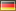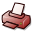# Machine learning in a setting of ordinal distance information

## DSpace Repository

 Dateien:

 URI: Dokumentart: Dissertation Date: 2017 Language: English Faculty: 7 Mathematisch-Naturwissenschaftliche Fakultät Department: Informatik Advisor: von Luxburg, Ulrike (Prof. Dr.) Day of Oral Examination: 2017-07-21 DDC Classifikation: 004 - Data processing and computer science Keywords: Maschinelles Lernen Other Keywords:ordinale Datenordinale Abstandsinformationordinal distance informationordinal data License: Order a printed copy:Print-on-Demand Show full item record

### Abstract:

In this thesis I make some contributions to the development of machine learning in a setting of ordinal distance information. A setting of ordinal distance information or ordinal data for short refers to the following scenario: The objects of interest are elements of a set X that is equipped with a dissimilarity function i, which quantifies how dissimilar two objects are. However, when given a data set of objects for which we want to solve a machine learning problem, we cannot evaluate i itself. Instead, we only get to see binary answers to some comparisons of dissimilarity values such as i(A,B)<i(C,D), where A,B,C,D are elements of the data set. Such a scenario has attracted interest in machine learning in recent years. It is in contrast to a scenario in which i can directly be evaluated and actual dissimilarity values i(A,B) are observed. The latter scenario is "standard" in machine learning and is referred to as a setting of cardinal distance information. My contributions are both on the theoretical and on the applied side. After the introductory Chapter 1, I present a result stating the asymptotic uniqueness of ordinal embeddings in Chapter 2. Constructing an ordinal embedding is the main approach to machine learning in a setting of ordinal distance information. The idea is to map the objects of the data set to points in a Euclidean space R^d such that the points preserve the given ordinal data as well as possible (with respect to the Euclidean interpoint distances). I show that for Euclidean data sets, which permit perfect ordinal embeddings, the points are uniquely determined up to a similarity transformation and small individual offsets that uniformly go to zero as the size of the data set goes to infinity. My result is the first of this kind in the literature and proves a long-standing claim dating back to the 1960s. In Chapter 3, I introduce two estimators for the intrinsic dimension of a data set that are based on only ordinal distance information. Although dimensionality estimation is a well-studied problem with a long history, all previous estimators from the literature are based on cardinal distance information. In Chapter 4 and Chapter 5, I provide algorithms for various machine learning problems in a setting of ordinal distance information. My algorithms do not construct an ordinal embedding of the data set, which would mean to transform the given ordinal data back to a "standard" cardinal setting. They rather directly make use of the ordinal data. In doing so, they avoid some of the drawbacks of an ordinal embedding approach. The algorithms that I propose in Chapter 4 are based on estimating the lens depth function or the k-relative neighborhood graph from ordinal distance information and are designed for specific machine learning problems. My algorithms of Chapter 5 yield positive-semidefinite kernel matrices on the data set and hence allow to apply any kernel method to the data set. They are the first generic means for solving machine learning problems in a setting of ordinal distance information in the literature that is different from the ordinal embedding approach.